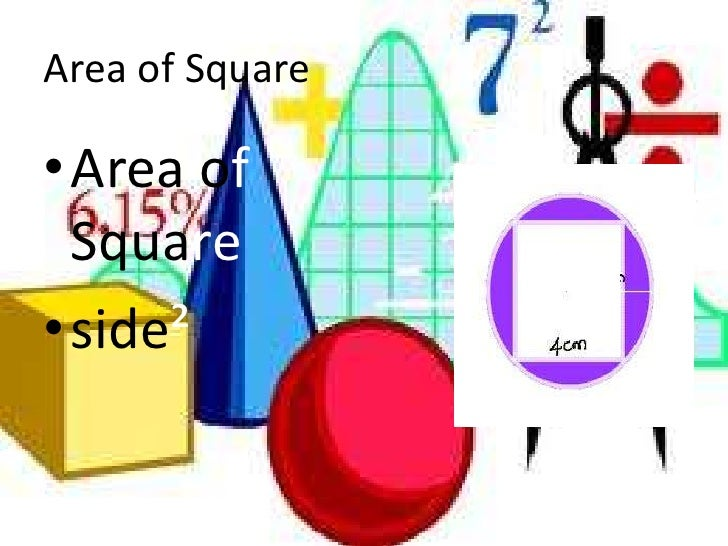# SSC CGL Quant Quiz MENSURATION – 18 Jul 20161. If the total surface area of a cube is 6 sq units, then what is the volume of the cube ?

1 cu unit
2 cu units
4 cu units
6 cu units
2. If the volume of a cube is 729 cu cm, what is the length of its diagonal ?
9√2 cm
9√3 cm
18 cm
18√3 cm

3. Find the surface area of a cuboid 10 m long, 5 m broad and 3 m high.

105 sq m
104 sq m
170 sq m
190 sq m

4. The surface area of a cube is 726 sq cm. Find the volume of the cube.

1331 cm3
1232cm3
1626cm3
1836 cm3

5. Three cubes of sides 1 cm, 6 cm and 8 cm are melted to form a new cube. Find half of the surface area of the new cube.

243 sq cm
463 sq cm
486 sq cm
293 sq cm

6. The maximum length of a pencil that can be kept in a rectangular box of dimensions 8 cm × 6 cm × 2 cm, is

2√13 cm
2√14 cm
2√26 cm
10√2 cm

7. Internal length, breadth and height of a rectangular box are 10 cm, 8 cm and 6 cm, respectively. How many boxes are needed which can be packed in a cube whose volume is 6240 cu. cm. ?

12
13
15
17

8. How many cubes of 3 cm edge can be cut out of a cube of 18 cm edge ?

63
432
216
None of these

9. A cube has each edge 2 cm and a cuboid is 1 cm long, 2 cm wide and 3 cm high. The paint in a certain container is sufficient to paint an area equal to 54 cm2. Which one of the following is correct ?

Both cube and cuboid can be painted
Only cube can be painted
Only cuboid can be painted
Neither cube nor cuboid can be painted

10. The whole surface area of a rectangular block is 8788 sq cm. If length, breadth and height are in the ratio of 4 : 3 : 2, then find the length.

26 cm
52 cm
104 cm
13 cm

11. The capacity of a cuboid tank of water is 50000 L. Find the breadth of the tank, if its length and depth are 2.5 m and 10 m, respectively.

2 m
4 m
9 m
6 m

12. The paint in certain container is sufficient to paint an area equal to 9.375 sq m. How many bricks of dimensions 22.5 cm × 10 cm × 7.5 cm can be painted out of this container?

100
200
50
175

13. What are dimensions (length, breadth and height, respectively) of a cuboid with volume 720 cu cm, surface area 484 sq cm and the area of the base 72 sq cm ?

9, 8 and 10 cm
12, 6 and 10 cm
18, 4 and 10 cm
30, 2 and 12 cm

14. The volume of a cube is numerically equal to sum of its edges. What is the total surface area in square units ?

12
36
72
144

15. If each side of a cube is decreased by 19%, then decrease in surface area is-

40%
38.4%
35%
34.39%

×

## Download success!

Thanks for downloading the guide. For similar guides, free study material, quizzes, videos and job alerts you can download the Adda247 app from play store.

Thank You, Your details have been submitted we will get back to you.

## Leave a comment

×
Login
OR

Forgot Password?

×
Sign Up
ORForgot Password
Enter the email address associated with your account, and we'll email you an OTP to verify it's you.Reset Password
Please enter the OTP sent to
/6

×
CHANGE PASSWORD